# How to Normalize and Standardize Data in R?

• Last Updated : 14 Jan, 2022

In this article, we will be looking at the various techniques to scale data,  Min-Max Normalization, Z-Score Standardization, and Log Transformation in the R programming language.

Let’s install and load the required packages. And also create a dataframe as a sample dataset.

## R

 `# load packages and data``install.packages``(``"caret"``)``library``(caret)` `# creating a dataset``data = ``data.frame``(var1=``c``(120, 345, 145, 122, 596, 285, 211),``                  ``var2=``c``(10, 15, 45, 22, 53, 28, 12),``                  ``var3=``c``(-34, 0.05, 0.15, 0.12, -6, 0.85, 0.11))` `data`

Output: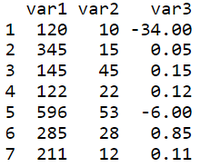## Summary of Data:

Let’s check out the summary of the data before scaling it. As we can see from the output, each variable/feature has a different range of values (which can be inferred from min and max values) and thus need scaling to bring the values within a fixed range.

## R

 `# import the library``library``(caret)` `# creating the dataset``data = ``data.frame``(var1 = ``c``(120,345,145,122,596,285,211),``           ``var2 = ``c``(10,15,45,22,53,28,12),``           ``var3 = ``c``(-34,0.05,0.15,0.12,-6,0.85,0.11))` `# summary of data``summary``(data)`

Output: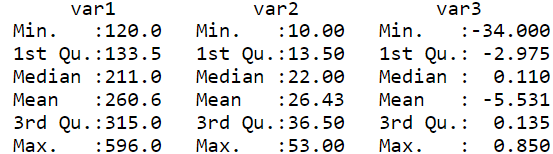## Normalization:

### Method 1: Min-Max Normalization

This technique rescales values to be in the range between 0 and 1. Also, the data ends up with smaller standard deviations, which can suppress the effect of outliers.

Example: Let’s write a custom function to implement Min-Max Normalization.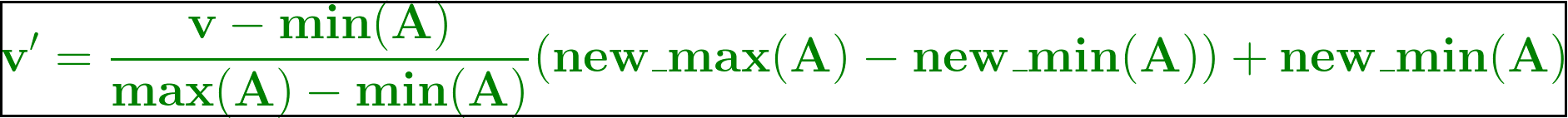Min-Max Normalization

This is the formula for Min-Max Normalization. Let’s use this formula and create a custom user-defined function, minMax which takes in one value at a time and computes the scaled value such that it lies between 0 and 1. Here new_max(A) is 1 and new_min(A) is 0 as we trying in scale down/up the values in the range [0,1].

This helps in handling the outliers well and suppresses them overall.

## R

 `# import the library``library``(caret)` `# dataset``data = ``data.frame``(var1 = ``c``(120,345,145,122,596,285,211),``           ``var2 = ``c``(10,15,45,22,53,28,12),``           ``var3 = ``c``(-34,0.05,0.15,0.12,-6,0.85,0.11))` `# custom function to implement min max scaling``minMax <- ``function``(x) {``  ``(x - ``min``(x)) / (``max``(x) - ``min``(x))``}` `#normalise data using custom function``normalisedMydata <- ``as.data.frame``(``lapply``(data, minMax))``head``(normalisedMydata)`

Output: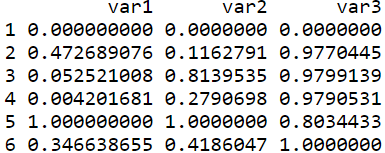Let’s now check if the values of the 4 columns are rescaled between 0 and 1 using a summary of the data (min and max are 0 and 1 respectively).

## R

 `# checking summary after normalization``summary``(normalisedMydata)`

Output: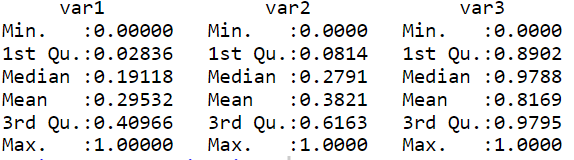Example: Using an in-built function and caret package to perform Min-Max Normalization

Here the method, preProcess( ) takes a tuple with value “range” to implement min-max scaling and this preprocessed data is sent to predict( ) function to get the final normalized data using the min-max scaling method.

Syntax:

preProcess(x, method = c(“center”, “scale”), … na.remove = TRUE )

Arguments:

• x – a matrix or data frame
• method – a character vector specifying the type of processing
• na.remove – true/false to specify removal of missing values

## R

 `# import the library``library``(caret)` `# dataset``data = ``data.frame``(var1 = ``c``(120,345,145,122,596,285,211),``           ``var2 = ``c``(10,15,45,22,53,28,12),``           ``var3 = ``c``(-34,0.05,0.15,0.12,-6,0.85,0.11))` `# preprocess the data``preproc <- ``preProcess``(mydata, method=``c``(``"range"``))` `# perform normalization``norm <- ``predict``(preproc, mydata)``head``(norm)`

Output:This technique tends to center the rescaled data around the mean, but it doesn’t handle outliers very well. So to tackle this we go for standardization.

### Method 2: Log Transformation

Not all real-life data would follow a gaussian distribution nor would be less skewed. So to tackle this Log Transformation technique can be used.

Example: Using log( ) function

Let’s log transform a particular column var2 in data and view it’s summary.

Syntax:

`log(x, base = exp(1))`

Arguments:

• x – a numeric or complex vector
• base – a positive or complex number

Log( ) function takes in numeric vector or complex vector of the data and performs log transformation.

## R

 `# import the library``library``(caret)` `# dataset``data = ``data.frame``(var1 = ``c``(120,345,145,122,596,285,211),``           ``var2 = ``c``(10,15,45,22,53,28,12),``           ``var3 = ``c``(-34,0.05,0.15,0.12,-6,0.85,0.11))` `# log transform on var2 column of data``logTransformed = ``log``(mydata\$var2)``logTransformed`

Output: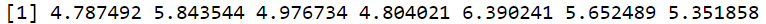Log Transformation

## Standardization:

Standardization is a technique in which all the features have a mean around zero and have roughly unit variance (mean = 0 and standard deviation = 1). And also makes sure that outliers get weighted more than other values.

Example : Using Standard scale( ) function

Function:

`scale(x, center = TRUE, scale = TRUE)`

Arguments:

• x – a numeric matrix(like object)
• center – either a logical value or numeric-alike vector of length equal to the number of columns of x
• scale – either a logical value or a numeric-alike vector of length equal to the number of columns of x

scale( ) function (a part of caret package in R) takes in a matrix or dataframe object and scales the data points such that the mean and standard deviation is 0 and 1 respectively.

## R

 `# import the library``library``(caret)` `# dataset``data = ``data.frame``(var1 = ``c``(120,345,145,122,596,285,211),``           ``var2 = ``c``(10,15,45,22,53,28,12),``           ``var3 = ``c``(-34,0.05,0.15,0.12,-6,0.85,0.11))` `# standardize the data using scale() function``standardizedData <- ``as.data.frame``(``scale``(data))``head``(standardizedData)`

Output: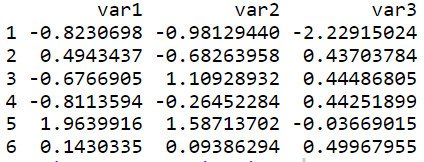Example: Using an in-built function in the caret library to preprocess and then standardize the data.

Here the method, preProcess( ) will take a tuple with values “center” and “scale” to implement standardization. This preprocessed data is sent to predict( ) to standardize the data such that the mean is 0 and the standard deviation is 1.

## R

 `# import the library``library``(caret)` `# dataset``data = ``data.frame``(var1 = ``c``(120,345,145,122,596,285,211),``           ``var2 = ``c``(10,15,45,22,53,28,12),``           ``var3 = ``c``(-34,0.05,0.15,0.12,-6,0.85,0.11))` `# using caret lib to preprocess data``preproc1 <- ``preProcess``(data, method=``c``(``"center"``, ``"scale"``))` `# standardize the preprocessed data``norm1 <- ``predict``(preproc1,data)``head``(norm1)`

Output:My Personal Notes arrow_drop_up